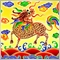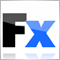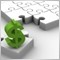36

Hi Guys

How do you add or subtract time in MQL4?

eg if I wanted to add or subtract 68 minutes to/from 06:04

Jay23883

Try this :

```      datetime TimeNow = TimeCurrent();
Print ( "TimeNow = ", TimeNow );
int AddNumber = 68; // in minute165200

1.) Change the time to be added or substracted to seconds

2.) change the time 6:04 from string to time format

3.) Carry out the operation

```int TimeToAdd=68*60; // change minutes to seconds

datetime NewTime=StrToTime("6:04")+TimeToAdd;   // Change time to datetime from string(hh:mm) format and carry out addition

string NewEasyToReadTime=TimeToStr(NewTime);    // Revert new time to string format of hh:mm:ss```[Deleted]

Hi there, How can i input time in hours on screen.

similar to c programming , scanf.....11732

MaryAnn Emmanuel:

Hi there, How can i input time in hours on screen.

similar to c programming , scanf.....

https://docs.mql4.com/dateandtime/hour

Hour - MQL4 Documentation
• docs.mql4.com
Hour - MQL4 Documentation[Deleted]
Thank you, it possible to time display on Screen and user keys in required time. example: (Time: user keys in desired time, example , 10.00).11732

MaryAnn Emmanuel:
Thank you, it possible to time display on Screen and user keys in required time. example: (Time: user keys in desired time, example , 10.00).
The easiest way to display it would be to use the Comment() function. Other ways would be with Text or Label objects. You could do the desired time in many ways, personally I'd give them a list of choices in a drop down menu in the inputs.
Comment - MQL4 Documentation
• docs.mql4.com
Comment - MQL4 Documentation10

mrjaywilliams:

Hi Guys

How do you add or subtract time in MQL4?

eg if I wanted to add or subtract 68 minutes to/from 06:04

Jay

int h=TimeHour(TimeCurrent());
int m=TimeMinute(TimeCurrent());
m=m+30;

string time = h+":"+m;

Print ( "Time = ", time );

This will work, in the above 30minutes have been added to current time.27851

Manoj Natarajan:
```int h=TimeHour(TimeCurrent());
int m=TimeMinute(TimeCurrent());
m=m+30;

string time = h+":"+m;

Print ( "Time = ", time );```

This will work, in the above 30minutes have been added to current time.

1. When you post code please use the CODE button (Alt-S)! (For large amounts of code, attach it.) Please edit your (original) post.
General rules and best pratices of the Forum. - General - MQL5 programming forum
Messages Editor

3. If the current time is 10:40 and you do m=m+30*60 you will display 10:70 "This will not work."10

William Roeder:
1. When you post code please use the CODE button (Alt-S)! (For large amounts of code, attach it.) Please edit your (original) post.
General rules and best pratices of the Forum. - General - MQL5 programming forum
Messages Editor

3. If the current time is 10:40 and you do m=m+30*60 you will display 10:70 "This will not work."

William, thank you for correcting me.

Am unable to edit the previous post.

few corrections made to previous code and now it will work

```int h = TimeHour(TimeCurrent());
int m = TimeMinute(TimeCurrent());    m=m+30;
int s = TimeSeconds(TimeCurrent());
string time,hh,mm,ss;
hh = h; mm = m; ss = s;

if (m > 59)
{
int t1 = m - 60;
mm = t1;
hh = h+1;
}

time = hh+":"+mm+":"+ss;
```27851

Manoj Natarajan: few corrections made to previous code and now it will work
1. nope. 23:40 + 30 minutes is not 24:10, it's 00:10.

2. Buy why not just add the 30 minutes and format the new datetime how you want it.

`string time = TimeToString(TimeCurrent() + 30*60, TIME_SECONDS);`